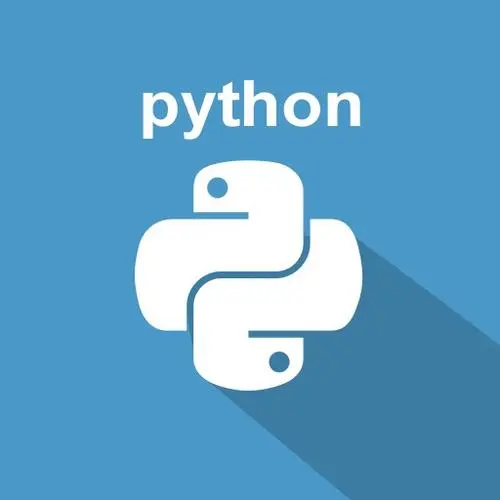趁着课余时间学点 Python（十二）面向对象的理解（结局）ベ布小禅@[toc]

1. 私有化

class Person(object):    def __init__(self, name, age):        self.xxx = name        self.xxxx = age

1.1 属性私有化

class Xxx(object):    age = 20    _name = "Xxx"

"""定义一个挺人类含有姓名，年龄，体重，身高将体重设置私有化通过类内部调用使得能够在类外部看到你的体重"""class Person(object):    _weight = 70    def __init__(self, name, age, height):        self.name = name        self.age = age        self.height = height     def weightPrint(self):        print("我的体重是：", self._weight)
person = Person("布小禅", 20, 180)person.weightPrint()

1.2 方法私有化

class Person(object):    def _love(self):        print("我暗恋Xxx")

class Person(object):    def _love(self):        print("我暗恋Xxx")        def speakLove(self):        self._love()

2. 重写

super().父类方法名(self)

class Person(object):    def __init__(self):        super().__init__(self)        print("我是重写加的")

3. 魔术方法

3.2 __str__方法

class Person(object):    def __str__(self):        return "我是__str__方法的返回值，显示在打印实例化对象时"
p = Person()print(p)

3.3 __del__方法

class Person(object):    def __del__(self):        print("我没用了，我被删除了")        p = Person()

3.4 __new__方法

class Person(object):    def __init__(self):        print("我是构造方法__init__")        def __new__(cls):        print("我是__new__方法")        p = Person()

class Person(object):    def __init__(self):        print("我是构造方法__init__")        def __new__(cls):        print("我是__new__方法")        return super().__new__(cls)    p = Person()

3.5 __call__方法

class Person(object):    def __call__(self):        print("执行了__call__方法")p = Person()print(p())

class Person(object):    def __call__(self):        print("执行了__call__方法")        return "我是返回值"p = Person()print(p())

结语评论